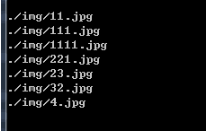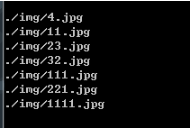# python文件排序都有哪些方法• 爱喝马黛茶的安东尼   2019-12-04 15:40:52486（1）首先：我测试的文件夹是/img/，里面的文件都是图片，如下图所示：（2）测试库函数sorted()，直接贴出代码：

```import numpy as np
import os

img_path='./img/'

img_list=sorted(os.listdir(img_path))#文件名按字母排序
img_nums=len(img_list)
for i in range(img_nums):
img_name=img_path+img_list[i]
print(img_name)```（3）测试函数sort()，代码：

```import numpy as np
import os
img_path='./img/'

img_list=os.listdir(img_path)
img_list.sort()
img_list.sort(key = lambda x: int(x[:-4])) ##文件名按数字排序
img_nums=len(img_list)
for i in range(img_nums):
img_name=img_path+img_list[i]
print(img_name)```python学习网，免费的在线学习python平台，欢迎关注！